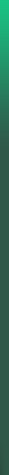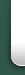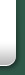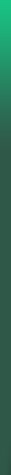Trailblazing Research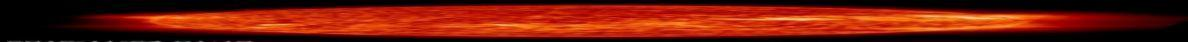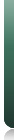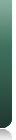HomeUnified Field Theory
 Unified Field Theory The Unified Field Theory was started by Einstein, but he was unable to complete it before he died.  The  theory attempts to unify all the forces of nature.  In honor of Einstein’s last request, “but my theories, they’re not finished”, Dr. Klein spent more than two decades developing a conceptual, graphical, and mathematical solution for the Unified Field Theory.     The original 4 forces of nature that were included in the Unified Field Theory  were: the strong nuclear force,  the weak nuclear force, electromagnetic force, and gravity. According to the Unified Field Theory, the forces of nature are not separate, but are all unified, or part of one more complex force (a unified field).  According to Dr. Klein’s Unifield Field Theory: Essentially, the unified field may be thought of as consisting of pairs of attractive and repulsive forces on different size scales.  There is a balance in nature;  thus, each attractive force  is balanced or opposed by a repulsive force.   For each size scale, there exists a pair of forces—an attractive force and a repulsive force that dominate at that size scale.  The dominant attractive force is balanced or opposed by the dominant repulsive force.  Thus, on the nuclear scale, there is the strong nuclear force (an attractive force that holds the protons and neutrons together in an atomic nucleus) and the weak nuclear force (which is responsible for nuclear decay). On a larger scale, there is the electromagnetic force, which has both attractive and repulsive components.  Thus, opposite charges attract each other, while like charges repel each other.  For magnetic fields, opposite poles attract each other, while like poles repel each other. On a still larger scale (from molecules to stars), gravity is the dominant attractive force, and entropy is a repulsive force that opposes gravity.  See the Gravity-Entropy theory for further explanation on gravity and entropy.  On a still larger scale, Dr. Klein postulated another Force, the Galactic Force.  The Galactic Force has an attractive component, which holds the stars together in a galaxy (gravity and the other forces of nature are too weak at the vast distances between stars).  The Galactic Force also has a repulsive component for balance, and is why the stars throughout the universe are grouped into galaxies, but are not on top of each other. The Galactic Force is also responsible for the motion of stars within a galaxy. On an even larger scale, Dr. Klein postulated the Galactic Cluster Force.  Hence, the Galactic Cluster Force causes galaxies throughout the universe to be grouped together in clusters.  As can be seen graphically below, all of these forces or fields are really part of one unified field.  In reality, since the  real universe is infinite, the field extends on even smaller scales, and also on even larger scales.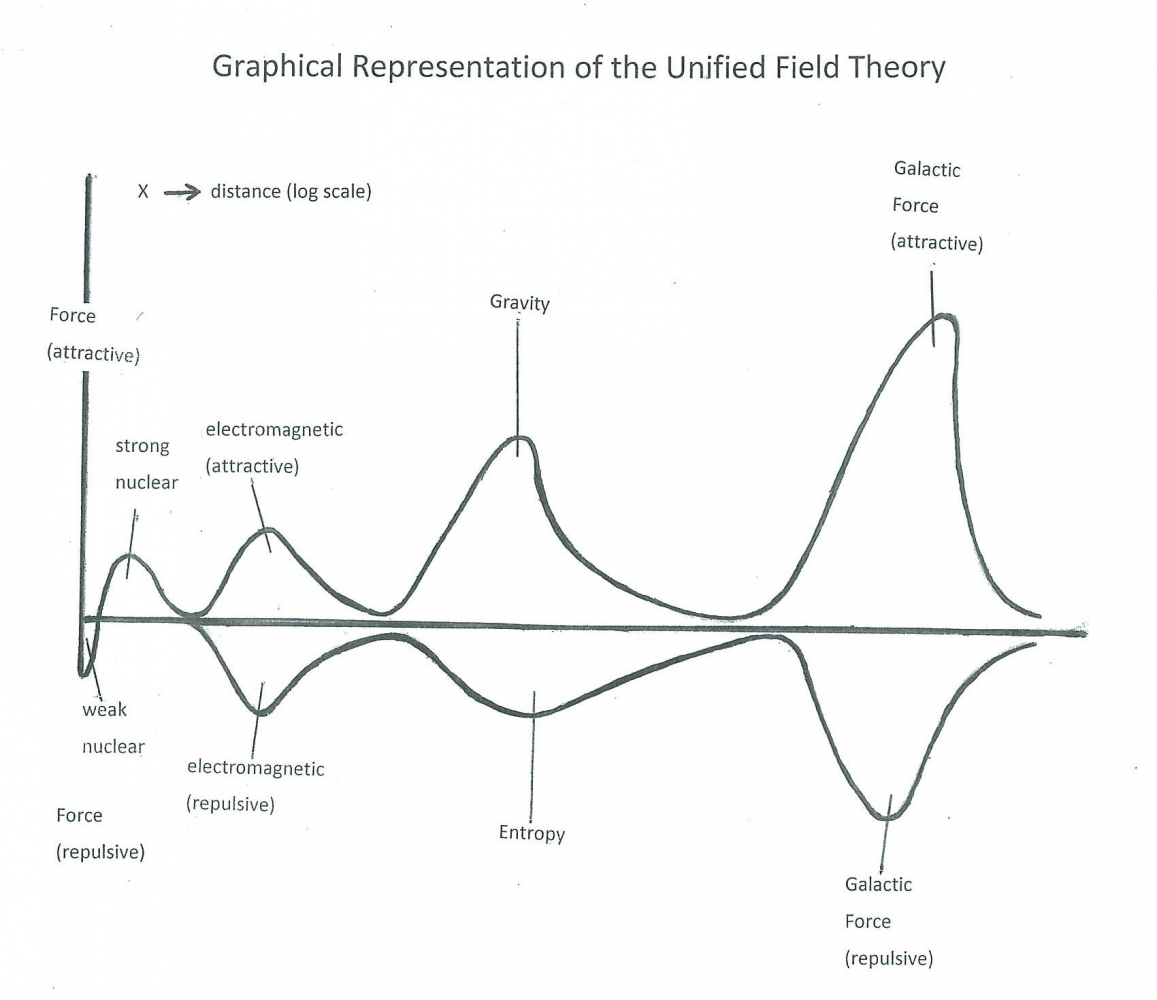Figure 4: Graphical Representation of the Unified Field Theory.  There is a balance in nature; for each attractive force, there is a corresponding repulsive force.  The forces of nature occur in attractive-repulsive force pairs on different size scales.  Each force creates a field.  As can be seen, the fields are all part of a larger, more complex unified field. This unified field can also be expressed mathematically.   The mathematical solution described by Dr. Klein is a system of parametric equations based on different size scales.  Thus, on a given size scale, for x greater than a, but less than b, a few equations dominate.  However, on a different size scale, for example, for X greater than b, but less than c, another set of equations dominate.  Further, it should be noted that the attractive forces are in a dynamic balance with the repulsive forces.  It is not a static balance.   The matter in the universe is constantly moving, constantly changing.